• matlab非线性函数拟合与实验报告（不使用工具箱）供新手参考使用的matlab代码，内含m文件与实验报告一份。
• 非线性拟合使用Matlab进行拟合是图像处理中线条变换的一个重点内容，本文将详解Matlab中的直线拟合和曲线拟合用法。关键函数：fittypeFit type for curve and surface fittingSyntaxffun = fittype(libname)ffun = ...
Matlab 线性拟合 & 非线性拟合使用Matlab进行拟合是图像处理中线条变换的一个重点内容，本文将详解Matlab中的直线拟合和曲线拟合用法。关键函数：fittypeFit type for curve and surface fittingSyntaxffun = fittype(libname)ffun = fittype(expr)ffun = fittype({expr1,…,exprn})ffun = fittype(expr, Name, Value,…)ffun= fittype({expr1,…,exprn}, Name, Value,…)/***********************************线性拟合***********************************/线性拟合公式：coeff1 * term1 + coeff2 * term2 + coeff3 * term3 + ...其中，coefficient是系数，term都是x的一次项。线性拟合Example：Example1: y=kx+b;法1：x=[1,1.5,2,2.5,3];y=[0.9,1.7,2.2,2.6,3];p=polyfit(x,y,1);x1=linspace(min(x),max(x));y1=polyval(p,x1);plot(x,y,‘*’,x1,y1);结果：p =    1.0200    0.0400即y=1.0200 *x+ 0.0400法2：x=[1;1.5;2;2.5;3];y=[0.9;1.7;2.2;2.6;3];p=fittype(‘poly1’)f=fit(x,y,p)plot(f,x,y);运行结果：x=[1;1.5;2;2.5;3];y=[0.9;1.7;2.2;2.6;3];p=fittype(‘poly1’)f=fit(x,y,p)plot(f,x,y);p =Linear model Poly1:p(p1,p2,x) = p1*x + p2f =Linear model Poly1:f(x) = p1*x + p2Coefficients (with 95% confidence bounds):p1 =        1.02  (0.7192, 1.321)p2 =        0.04  (-0.5981, 0.6781)Example2:y=a*x + b*sin(x) + c法1：x=[1;1.5;2;2.5;3];y=[0.9;1.7;2.2;2.6;3];EXPR = {‘x’,‘sin(x)’,‘1’};p=fittype(EXPR)f=fit(x,y,p)plot(f,x,y);运行结果：x=[1;1.5;2;2.5;3];y=[0.9;1.7;2.2;2.6;3];EXPR = {‘x’,‘sin(x)’,‘1’};p=fittype(EXPR)f=fit(x,y,p)plot(f,x,y);p =Linear model:p(a,b,c,x) = a*x + b*sin(x) + cf =Linear model:f(x) = a*x + b*sin(x) + cCoefficients (with 95% confidence bounds):a =       1.249  (0.9856, 1.512)b =      0.6357  (0.03185, 1.24)c =     -0.8611  (-1.773, 0.05094)法2：x=[1;1.5;2;2.5;3];y=[0.9;1.7;2.2;2.6;3];p=fittype(‘a*x+b*sin(x)+c’,‘independent’,‘x’)f=fit(x,y,p)plot(f,x,y);运行结果：x=[1;1.5;2;2.5;3];y=[0.9;1.7;2.2;2.6;3];p=fittype(‘a*x+b*sin(x)+c’,‘independent’,‘x’)f=fit(x,y,p)plot(f,x,y);p =General model:p(a,b,c,x) = a*x+b*sin(x)+cWarning: Start point not provided, choosing random startpoint.> In fit>iCreateWarningFunction/nThrowWarning at 738In fit>iFit at 320In fit at 109f =General model:f(x) = a*x+b*sin(x)+cCoefficients (with 95% confidence bounds):a =       1.249  (0.9856, 1.512)b =      0.6357  (0.03185, 1.24)c =     -0.8611  (-1.773, 0.05094)/***********************************非线性拟合***********************************/Example：y=a*x^2+b*x+c法1：x=[1;1.5;2;2.5;3];y=[0.9;1.7;2.2;2.6;3];p=fittype(‘a*x.^2+b*x+c’,‘independent’,‘x’)f=fit(x,y,p)plot(f,x,y);运行结果：p =General model:p(a,b,c,x) = a*x.^2+b*x+cWarning: Start point not provided, choosing random startpoint.> In fit>iCreateWarningFunction/nThrowWarning at 738In fit>iFit at 320In fit at 109f =General model:f(x) = a*x.^2+b*x+cCoefficients (with 95% confidence bounds):a =     -0.2571  (-0.5681, 0.05386)b =       2.049  (0.791, 3.306)c =       -0.86  (-2.016, 0.2964)法2：x=[1;1.5;2;2.5;3];y=[0.9;1.7;2.2;2.6;3];%use c=0;c=0;p1=fittype(@(a,b,x) a*x.^2+b*x+c)f1=fit(x,y,p1)%use c=1;c=1;p2=fittype(@(a,b,x) a*x.^2+b*x+c)f2=fit(x,y,p2)%predict cp3=fittype(@(a,b,c,x) a*x.^2+b*x+c)f3=fit(x,y,p3)%show resultsscatter(x,y);%scatter pointc1=plot(f1,‘b:*’);%bluehold onplot(f2,‘g:+’);%greenhold onplot(f3,‘m:*’);%purplehold off喜欢 (0)or分享 (0)
展开全文• 深度学习 神经网络 BP 多层感知机 非线性函数 拟合 matlab
• BP神经网络的非线性系统建模_非线性函数拟合matlab代码，可以直接运行！
• MATLAB 实现BP神经网络的非线性系统建模-非线性函数拟合BP神经网络
• 前两天练题的时候要用到多元非线性拟合，但是在网上找到的大多都是一些求问的帖子，真正有用的回答却不多，这里汇总一下与大家分享。 首先导入数据（参数和结果） 确定自己要拟合函数（自己画个散点图预估一下，...
Process
前两天练题的时候要用到多元非线性拟合，但是在网上找到的大多都是一些求问的帖子，真正有用的回答却不多，这里汇总一下与大家分享。

首先导入数据（参数和结果）
确定自己要拟合的函数（自己画个散点图预估一下，或者是使用SPSS的曲线估算，但是没自己画图看的舒服）
确定要拟合的系数的预估值（全部写零也行，但是你得有个大概想法，比如你图像是幂次为负的幂函数，那么你就写个负值，比如-1）
套用nlinfit函数，得出的bate矩阵就是你要拟合的系数矩阵。
covB回归系数的协方差
mse均方误差
beta参数最优值
r各点处的拟合残差
J雅各比矩阵的数值
ErrorModelInfo错误模型信息

Code
clear all;
a=xlsread("C:\Users\123\Desktop\数据.xlsx","a");%导入表a
b=xlsread("C:\Users\123\Desktop\数据.xlsx","b");%导入表b
s=a(:, 6);%y值(结果矩阵)
myfunc=inline('beta(1).*x(:,1).^(beta(2))+beta(3).*x(:,2).^(beta(4))+beta(5).*x(:,3).^(beta(6))+beta(7).*x(:,4).^(beta(8))+beta(9)','beta','x');%内联函数（beta是函数自带的代表待确定的系数）
beta0 = [1, -2 ,1, -2 ,1, -2 ,1, -2 ,60];%待定系数的预估值
[beta,R,J,CovB,MSE,ErrorModelInfo]= nlinfit(b, s, myfunc,beta0);
beta



展开全文• 本资源是BP神经网络的非线性系统建模-非线性函数拟合matlab仿真，分析了基于BP神经网络的非线性函数拟合算法，算法流程分为BP神经网络构建、BP神经网络训练和BP神经网络预测。
• matlab经典智能算法案例分析-BP神经网络的非线性系统建模-非线性函数拟合
• 点击上方蓝字关注“公众号”MATLAB BP神经网络的非线性系统建模-非线性函数拟合在实际工程应用中会遇到一些复杂的非线性系统，这些系统方程复杂，难以用数学方法建模。在这种情况下，可以使用BP神经网络表达这些非...


点击上方蓝字关注“公众号”MATLAB  BP神经网络的非线性系统建模-非线性函数拟合在实际工程应用中会遇到一些复杂的非线性系统，这些系统方程复杂，难以用数学方法建模。在这种情况下，可以使用BP神经网络表达这些非线性系统。该方法把位置系统看成是一个黑箱，首先用系统输入输出数据训练BP神经网络，使网络能够表达该未知函数，然后用训练好的BP神经网络预测系统输出。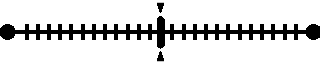基于BP神经网络的非线性函数拟合算法流程可以分为BP神经网络构建、BP神经网络训练和BP神经网络预测三步。 BP神经网络构建根据拟合非线性函数特点确定BP神经网络结构，由于该非线性函数有两个输入参数，一个输出参数，所以BP神经网络结构为2-5-1，即输入层有两个节点，隐含层有5个节点，输出层有1个节点。BP神经网络训练用非线性函数输入输出数据训练神经网络，使训练后的网络能够预测非线性函数输出。从非线性函数中随机得到2000组输入输出数据，从中随机选择1900组作为训练数据，用于网络训练，100组作为测试数据，用于测试网络的训练性能。神经网络预测用训练好的网络预测函数输出，并对预测结果进行分析。根据非线性函数方程随机得到该函数的2000组输入输出数据，将数据存储在data.mat文件中，input是函数输入数据，output是函数输出数据。从输入输出数据中随机选取1900组数据作为网络训练数据，100组数据作为网络测试数据，并对训练数据进行归一化处理。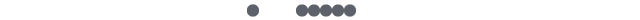壹01主程序介绍%% 清空环境变量clcclear%% 训练数据预测数据提取及归一化%下载输入输出数据load data input output%从1到2000间随机排序k=rand(1,2000);[m,n]=sort(k);%找出训练数据和预测数据input_train=input(n(1:1900),:)';output_train=output(n(1:1900));input_test=input(n(1901:2000),:)';output_test=output(n(1901:2000));贰01仿真主程序%选连样本输入输出数据归一化[inputn,inputps]=mapminmax(input_train);[outputn,outputps]=mapminmax(output_train);%% BP网络训练% %初始化网络结构net=newff(inputn,outputn,5);net.trainParam.epochs=100;net.trainParam.lr=0.1;net.trainParam.goal=0.00004;%网络训练net=train(net,inputn,outputn);02%% BP网络预测%预测数据归一化inputn_test=mapminmax('apply',input_test,inputps);%网络预测输出an=sim(net,inputn_test);%网络输出反归一化BPoutput=mapminmax('reverse',an,outputps);03%网络预测输出an=sim(net,inputn_test);%网络输出反归一化BPoutput=mapminmax('reverse',an,outputps);%% 结果分析figure(1)plot(BPoutput,':og')hold onplot(output_test,'-*');legend('预测输出','期望输出')title('BP网络预测输出','fontsize',12)ylabel('函数输出','fontsize',12)xlabel('样本','fontsize',12)%预测误差error=BPoutput-output_test;04仿真结果图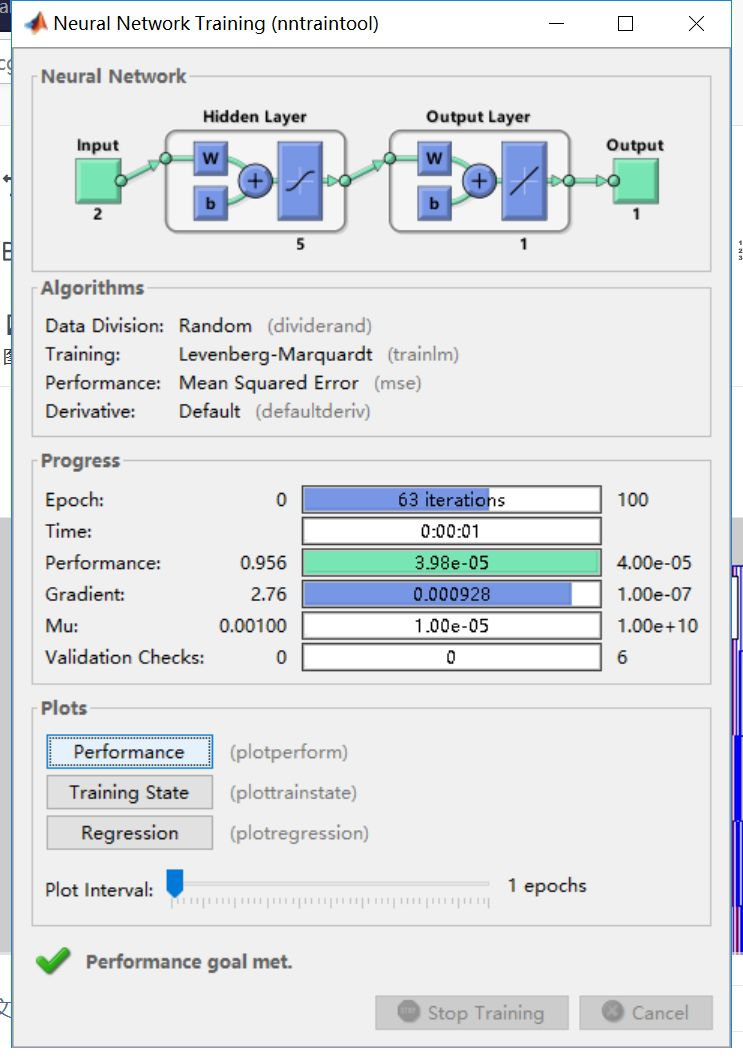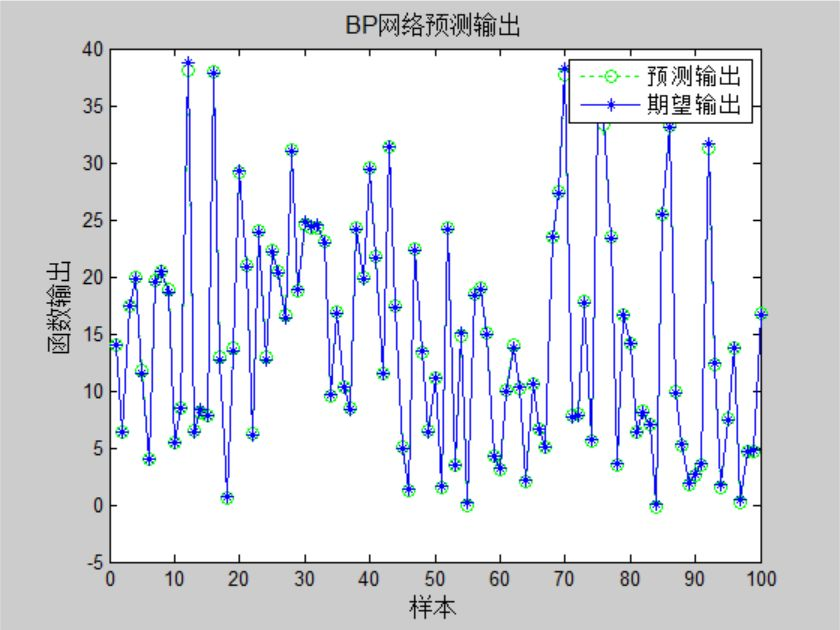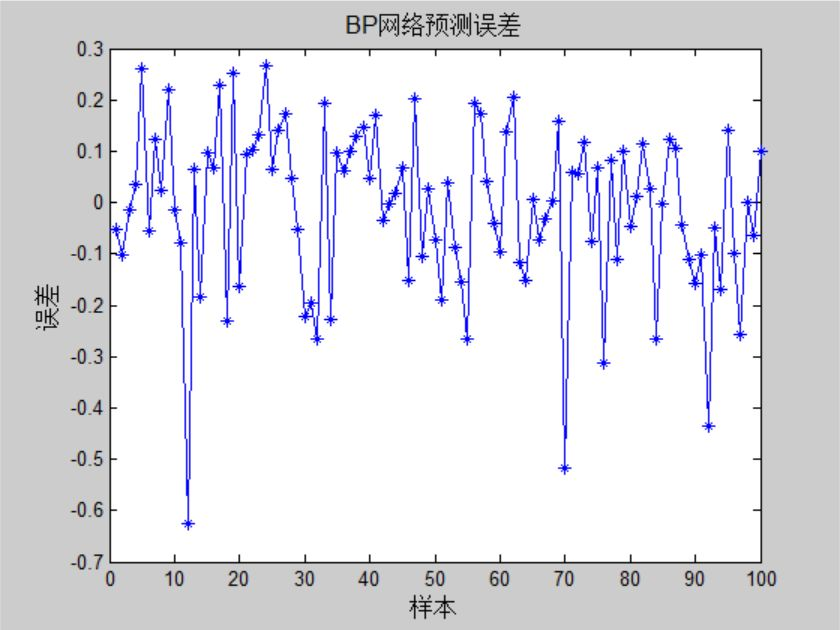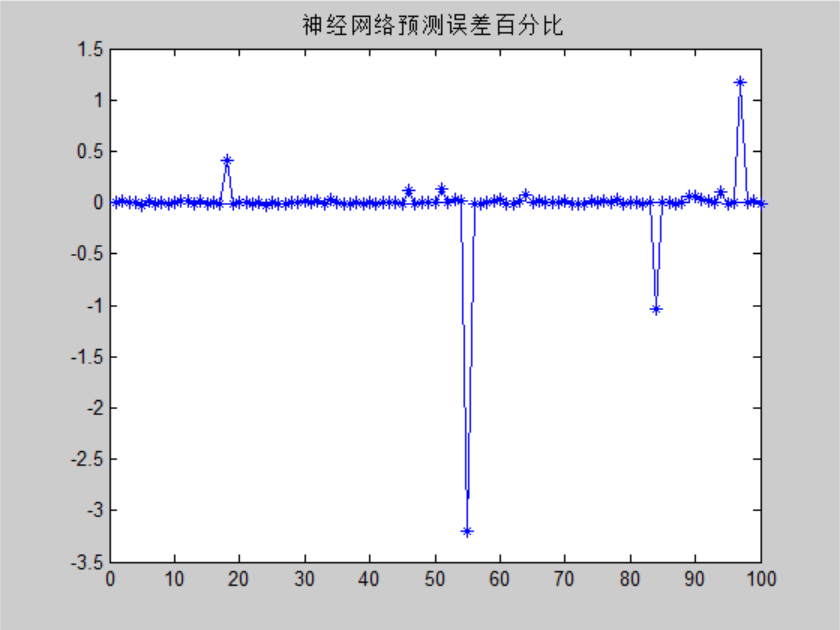具体仿真程序链接，微信公众号回复【神经网络】即可获得链接。未完待续扫码关注不迷路展开全文• 点击上方蓝字关注“公众号”MATLAB BP神经网络的非线性系统建模-非线性函数拟合在实际工程应用中会遇到一些复杂的非线性系统，这些系统方程复杂，难以用数学方法建模。在这种情况下，可以使用BP神经网络表达这些非...


点击上方蓝字关注“公众号”MATLAB  BP神经网络的非线性系统建模-非线性函数拟合在实际工程应用中会遇到一些复杂的非线性系统，这些系统方程复杂，难以用数学方法建模。在这种情况下，可以使用BP神经网络表达这些非线性系统。该方法把位置系统看成是一个黑箱，首先用系统输入输出数据训练BP神经网络，使网络能够表达该未知函数，然后用训练好的BP神经网络预测系统输出。基于BP神经网络的非线性函数拟合算法流程可以分为BP神经网络构建、BP神经网络训练和BP神经网络预测三步。 BP神经网络构建根据拟合非线性函数特点确定BP神经网络结构，由于该非线性函数有两个输入参数，一个输出参数，所以BP神经网络结构为2-5-1，即输入层有两个节点，隐含层有5个节点，输出层有1个节点。BP神经网络训练用非线性函数输入输出数据训练神经网络，使训练后的网络能够预测非线性函数输出。从非线性函数中随机得到2000组输入输出数据，从中随机选择1900组作为训练数据，用于网络训练，100组作为测试数据，用于测试网络的训练性能。神经网络预测用训练好的网络预测函数输出，并对预测结果进行分析。根据非线性函数方程随机得到该函数的2000组输入输出数据，将数据存储在data.mat文件中，input是函数输入数据，output是函数输出数据。从输入输出数据中随机选取1900组数据作为网络训练数据，100组数据作为网络测试数据，并对训练数据进行归一化处理。壹01主程序介绍%% 清空环境变量clcclear%% 训练数据预测数据提取及归一化%下载输入输出数据load data input output%从1到2000间随机排序k=rand(1,2000);[m,n]=sort(k);%找出训练数据和预测数据input_train=input(n(1:1900),:)';output_train=output(n(1:1900));input_test=input(n(1901:2000),:)';output_test=output(n(1901:2000));贰01仿真主程序%选连样本输入输出数据归一化[inputn,inputps]=mapminmax(input_train);[outputn,outputps]=mapminmax(output_train);%% BP网络训练% %初始化网络结构net=newff(inputn,outputn,5);net.trainParam.epochs=100;net.trainParam.lr=0.1;net.trainParam.goal=0.00004;%网络训练net=train(net,inputn,outputn);02%% BP网络预测%预测数据归一化inputn_test=mapminmax('apply',input_test,inputps);%网络预测输出an=sim(net,inputn_test);%网络输出反归一化BPoutput=mapminmax('reverse',an,outputps);03%网络预测输出an=sim(net,inputn_test);%网络输出反归一化BPoutput=mapminmax('reverse',an,outputps);%% 结果分析figure(1)plot(BPoutput,':og')hold onplot(output_test,'-*');legend('预测输出','期望输出')title('BP网络预测输出','fontsize',12)ylabel('函数输出','fontsize',12)xlabel('样本','fontsize',12)%预测误差error=BPoutput-output_test;04仿真结果图具体仿真程序链接，微信公众号回复【神经网络】即可获得链接。未完待续扫码关注不迷路展开全文bp神经网络预测
• 遗传算法优化BP神经网络-非线性函数拟合 matlab程序
• 本图文详细介绍了如何利用Matlab神经网络工具箱实现BP神经网络对非线性函数拟合神经网络 BP神经网络 newff
• 本资源是遗传算法优化BP神经网络-非线性函数拟合matlab仿真程序，遗传算法的基本要素包括染色体编码方法、适应度函数、遗传操作和运行参数。
• 本图文详细介绍了如何利用Matlab神经网络工具箱实现多层BP神经网络对非线性函数拟合神经网络 多层BP神经网络 newff
• model:事先用M文件定义的非线性函数 beta0：回归系数的初始值 定义列表语法2： [Y, Delta]=nlpredci(‘model’,x,beta,r,J) 用于求取x对应的预测值Y，以及在alpha下的置信区间Delta。 代码块 ...nlinfit
• 采用matlab语言编写的遗传算法优化BP神经网络，并以非线性函数拟合为例评价适用性。
• 函数功能：高斯牛顿法实现非线性数据拟合。 代码 clear;clc; M=1000; Te=20; % 模型 t=Te*(1:M)'; load noise; % 噪音可自己添加 Et1=0.4*exp(-t/50)+0.6*exp(-t/200); Et2=0.4*exp(-(t/50).^2)+0.6*exp(-t/200); ...
• 遗传算法优化BP神经网络---非线性函数拟合,matlab遍的遗传算法
• 在科学计算和工程应用中，经常会遇到需要拟合一系列的离散数据，最近找了很多相关的文章方法，在这里进行总结一下其中最完整、几乎能解决所有离散参数非线性拟合的方法 1 得到散点数据 根据你的实际问题得到一系列的...
• matlab-非线性拟合函数lsqcurvefit的使用和初值选取 所解决问题： 我们知道我们的表达式是y=A+B*exp(-x.^2)-C./log(x), 而且现在我们手里面有x与y对应的一大把数据。 我们需要根据x, y的值找出最佳...
• 本微信图文详细介绍了遗传算法优化BP神经网络初始权值阈值的过程，并通过实例说明该优化能够提升BP神经网络的预测精确程度。神经网络 遗传算法 GA优化BP BP神经网络
• ## matlab lsqcurvefit 非线性拟合

万次阅读 多人点赞 2018-08-19 20:28:35
非线性曲线拟合是已知输入向量xdata和输出向量ydata，并且知道输入与输出的函数关系为ydata=...拟合如下非线性函数 函数曲线如下所示。 fit_fun.m function diff = fit_fun(param, X) a = param(1); m = pa...
• 文章目录[MATLAB 在科学计算中的应用] 使用MATLAB 进行非线性拟合前言引述MATLAB 曲线拟合函数简述一二维数据非线性拟合一维数据拟合例子二维数据拟合例子高维数据非线性拟合lsqcurvefitnlinfit 函数数据拟合工具箱...
• matlab进行非线性拟合常用最小二乘法，适用于：已经求解出函数，但含有未知数，不过已经收集到了一系列数据 1.lsqcurvefit 格式：[x, resnorm,r,flag]=lsqcurvefit(fun, c0,xdata,ydata) c0为初始解向量；xdata，......

# matlab非线性函数拟合matlab 订阅